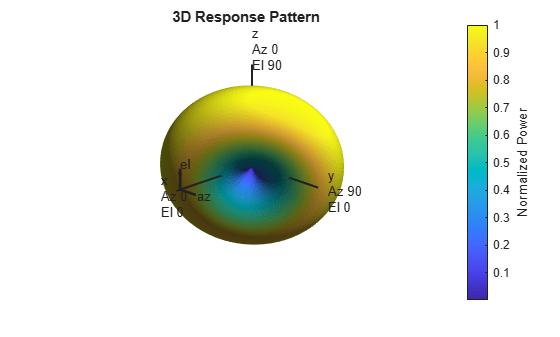# rotpat

## Syntax

``rpat = rotpat(pat,az,el,rotax)``
``rpat = rotpat(pat,az,el,rotax,expval)``

## Description

example

````rpat = rotpat(pat,az,el,rotax)` rotates a radiation pattern, `pat`, into a new pattern, `rpat`, whose boresight is aligned with the x-axis of a new local coordinate system defined by `rotax`. `az` and `el` specify the azimuth and elevation angles at which the original pattern is sampled.```
````rpat = rotpat(pat,az,el,rotax,expval)` also specifies an extrapolated value to be used when `az` and `el` do not cover the entire 3-D space. TipYou can use this function to rotate real and complex scalar radiation patterns as well as the orthogonal components of polarized fields. To rotate polarized fields, rotate the horizontal and vertical polarization components separately. ```

## Examples

collapse all

Use a short-dipole antenna to create a polarized radiation pattern. Rotate the pattern and use the rotated pattern as the radiation pattern of a custom antenna.

Create a `phased.ShortDipoleAntennaElement `antenna object with default properties. The short-dipole antenna radiates polarized radiation. Obtain and display the radiation for all directions.

```antenna1 = phased.ShortDipoleAntennaElement; el = -90:90; az = -180:180; pat_h = zeros(numel(el),numel(az),'like',1+1i); pat_v = pat_h; fc = 3e8; for m = 1:numel(el) temp = antenna1(fc,[az;el(m)*ones(1,numel(az))]); pat_h(m,:) = temp.H; pat_v(m,:) = temp.V; end pattern(antenna1,fc,'Type','Power')```Rotate the antenna pattern around the y-axis by 135 degrees followed by a rotation around the x-axis by 65 degrees.

```newax = rotx(65)*roty(135); pat2_h = rotpat(pat_h,az,el,newax); pat2_v = rotpat(pat_v,az,el,newax);```

Insert the rotated pattern into a `phased.CustomAntennaElement` object. Set the antenna polarization properties so that the element radiates horizontal and vertical polarized fields. Then display the rotated pattern in three dimensions.

```antenna2 = phased.CustomAntennaElement( ... 'SpecifyPolarizationPattern',true, ... 'HorizontalMagnitudePattern',mag2db(abs(pat2_h)), ... 'HorizontalPhasePattern',rad2deg(angle(pat2_h)), ... 'VerticalMagnitudePattern',mag2db(abs(pat2_v)), ... 'VerticalPhasePattern',rad2deg(angle(pat2_v))); pattern(antenna2,fc,'Type','Power')```Create a radiation pattern for a cosine antenna using a `phased.CosineAntennaElement` object. Rotate the pattern to use in a `phased.CustomAntennaElement` antenna object.

First obtain the radiation pattern for a `phased.CosineAntennaElement` object over a limited range of directions. The field is not polarized.

```antenna1 = phased.CosineAntennaElement('CosinePower',[5,5]); az = -60:65; el = -60:60; pat = zeros(numel(el),numel(az),'like',1); fc = 300e6; for m = 1:numel(el) temp = antenna1(fc,[az;el(m)*ones(1,numel(az))]); pat(m,:) = temp; end```

Display the original pattern.

```imagesc(az,el,abs(pat)) axis xy axis equal axis tight xlabel('Azimuth (deg)') ylabel('Elevation (deg)') title('Original Radiation Pattern') colorbar```Rotate the antenna pattern by 20 degrees around the z-axis and 50 degrees around the x-axis. Then display the rotated pattern.

```newax = rotx(50)*rotz(20); rpat = rotpat(pat,az,el,newax); imagesc(az,el,abs(rpat)) axis xy axis equal axis tight xlabel('Azimuth (deg)') ylabel('Elevation (deg)') title('Rotated Radiation Pattern') colorbar```Use the rotated pattern in a custom antenna element and display the pattern in 3-D.

```antenna2 = phased.CustomAntennaElement( ... 'AzimuthAngles',az,'ElevationAngles',el,'SpecifyPolarizationPattern',false, ... 'MagnitudePattern',mag2db(abs(rpat)), ... 'PhasePattern',zeros(size(rpat))); pattern(antenna2,fc,'Type','Power')```## Input Arguments

collapse all

Radiation pattern, specified as a complex-valued N-by-M matrix or complex-valued N-by-M-by-L array. N is the length of the `el` vector and M is the length of the `az` vector. Each column corresponds to one of the azimuth angles specified in the `az` argument. Each row corresponds to one of the elevation angles specified in the `el` argument. You can specify multiple radiation patterns using L pages. For example, you can use pages to specify radiation patterns at different frequencies. The main lobe of each pattern is assumed to point along the x-axis. Units are in meters-squared.

Data Types: `double`
Complex Number Support: Yes

Azimuth angles for computing 3-D radiation pattern, specified as a 1-by-M real-valued row vector where M is the number of azimuth angles. Each entry corresponds to one of the columns of the matrix specified in the `pat` argument. Angle units are in degrees. Azimuth angles must lie between –180° and 180°, inclusive.

The azimuth angle is the angle between the x-axis and the projection of the direction vector onto the xy-plane. The azimuth angle is positive when measured from the x-axis toward the y-axis.

Example: `-45:2:45`

Data Types: `double`

Elevation angles for computing directivity and pattern, specified as a 1-by-N real-valued row vector where N is the number of elevation angles. Each entry corresponds to one of the rows of the matrix specified in the `pat` argument. Angle units are in degrees. Elevation angles must lie between –90° and 90°, inclusive.

The elevation angle is the angle between the direction vector and xy-plane. The elevation angle is positive when measured toward the z-axis.

Example: `-75:1:70`

Data Types: `double`

Rotation matrix, specified as a real-valued orthonormal 3-by-3 matrix or a real-valued 3-by-3-by-P array. The columns represent the x, y, and z directions of the rotated coordinate system with respect to the original coordinate system. The P pages specify different rotation matrices.

This table describes how dimensions of the output pattern `rpat` depend on the dimensions of the `pat` and `rotax` arguments.

Dimensions of `rpat`

`Dimensions of pat````Dimensions of rotax```
3-by-33-by-3-by-P
M-by-NRotate a single pattern by a single rotation matrix. Output dimensions of `rpat` are M-by-N.Rotate a single pattern by P different rotation matrices. Output dimensions of `rpat` are M-by-N-by-P.
M-by-N-by-LRotate L patterns by the same rotation matrix. Output dimensions of `rpat` are M-by-N-by-L.In this case, P must equal L and the function rotates each pattern by the corresponding rotation matrix. Output dimensions of `rpat` are M-by-N-by-L.

Example: `rotx(45)*roty(30)`

Data Types: `double`

Extrapolation value, specified as a scalar. This scalar is the extrapolated value when the rotated patterns do not fill the entire 3-D space specified by `az` and `el`. In general, consider setting `expval` to 0 if the pattern is specified in a linear scale or `-inf` if the pattern is specified in a dB scale.

Example: `-inf`

Data Types: `double`

## Output Arguments

collapse all

Rotated radiation pattern, returned as a complex-valued N-by-M matrix or complex-valued N-by-M-by-P array. N is the length of the `el` vector. M is the length of the `az` vector. The dimensionality of `pat` and `rotax` determine the value of P as discussed in the `rotax` input argument. Units are in meters-squared.

Data Types: `double`
Complex Number Support: Yes

## Version History

Introduced in R2019a